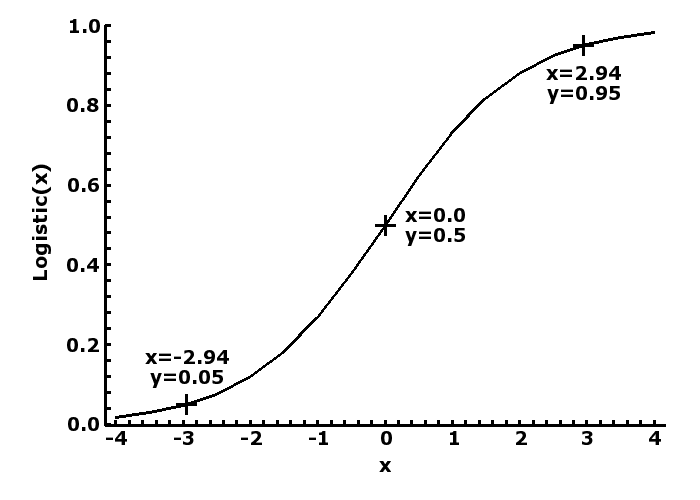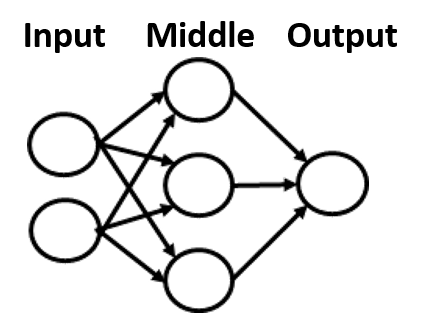Content DisclaimerCopyright @2020.All Rights Reserved.
StatsToDo : BackPropagation Neural Network Explained and Program
 Introduction Program This page provides the program and explanations for the basic Backpropagation Neural Net. Neural net is a vast subject, and subjected to rapid development in the 21st century, as it forms the basis of machine learning and artificial intelligence, and backprpagation is one of the earliest to develop, and form the basic framework for many algorithms. Backpropagation began as a simple adaptive learning algorithm, and this is presented in this page, in the form of a Javascript program, so that users can use the program directly, or if required, copy and adapt the algorithm into their own programs. The program is best viewed as a form of non-parametric regression, where the variables are based on Fuzzy Logic, a number between 0 (false) and 1 (true). Fuzzy Logic The Greek philosopher, Aristotle, stated that things can be true or not true, but cannot be both. Fuzzy logic replaces this statement with that true and false are only extremes that seldom exists, while reality is mostly somewhere in between.Mathematically this is represented as a number (y) between 0 (false) and 1 (true), and its relationship to a linear measurement (x) represented by the logistic curve (y = 1/(1+exp(-x)), as shown in the plot to the left, where a value of 0 is translated to a probability of 0.5, -∞ to 0 and or +∞ to 1. If we then accept that <=0.05 as unlikely to be true and >=0.95 likely to be true, then we can rescale any measurement to -2.9444 and + 2.9444, which is then logistically transformed to 0.05 and 0.95. Program for logistic transformation is available Numerical Transformation Program Page .An example of this is shown in the plot to the right, which translates the measurement of fetal blood pH into a diagnosis of acidosis, by firstly rescale the normally accepted non-acidosis value of 7.35 to -2.4444 and its logistic value of 0.05 and the normally accepted acidosis value of 7.2 to 2.4444 and its logistic value of 0.95. This rescaling changes an otherwise normally distributed measurement into the bimodal one of acidosis and non-acidosis, compressing the values less than 7.2 and more then 7.35, while stretches the values in between. Neurone The processing unit in a Backpropagation neuronet is the perceptron, based on the concept of the nerve cell the neurone. The unit receives one or more inputs (dendrites), process them to produce an output (axon). Mathematically, this is divided into two processes. The first is to combined the inputs so the y= Σwivi + c, where v are input values, w the weights given to each input, and c the bias value The combined value (y) is then transformed into a Fussy Logic value between 0 (false) and 1 (true). This can be binary (>0.5=1, <0.5=0), but most commonly the logistic transform is used. Neuronet and BackpropagationThe Backpropagation neuronet is an arrangement of neurones as shown to the right, and consists of the following The input layer, which contains as many neurone as there are inputs. In this example, there are 2 input neurones One or more middle layer, each containing a number of neurones. In this example there is 1 middle layer containing 3 neurones The output layer, which contains as many neurones as there are outputs. In this example, there is 1 output neurone Tranining the neuronet The coefficients (w and c) in all of the neurones in a backpropagation neuronet consist of random numbers when the neuronet is initially constructed. Training consists of presenting a series of templates (input and output) to the neuronet, which adapts (learn) through the following processes Forward Propagation Each input entered via the input layer is entered into each neurone of the middle layer. Each neurone then processes all the inputs (dendrite) and produces its output (axon) If there is more than 1 layer, the outputs from each layer becomes the inputs of the next layer, until the output layer, the neurones of which produce the final output values. Backward Propagation The output values are compared with the template output values. The coefficients in each neurone (w and c) are then changed so that results would be closer to the template output values Going backwords through the layers, eack preceeding layer is similarly altered so that the output from each neurone would produce an output that is closer to the required value For each template in the training data set, the error produced is estimated and compared with the output values in the template The maximum error for each iteration of the whole dataset is estimated, and compared with the acceptable error value. The training is re-iterated until the maximum error for each iteration is less than the acceptable error. At this point, the training is completed, and the values in the coefficients represent the "memory" of the training, and can be used to reproduce the template output values from inputs. Using the trained neuronet At the end of training, the set of coefficients represents the "memory" that has been trained, and can be use to produce outputs from sets of input. Simple neuronet can be process manually, but usually the set of coefficients is incorporated into a computer program or hardwired into machineries. References Users should be aware that neural network generally, and backpropagation in particular, have undergone dramatic development in the 21st century, and the current complexity and capability of these algorithms greatly exceed the content of this page. The program on this page is a simple and primitive one, and can probably used for diagnostic or therapeutic decision making in clearly defined clinical domains, with 5-20 inputs,10-20 patterns to learn, and training dataset of no more than a few hundred templates. It is insufficient to process complex patterns that requires large datasets such as in predicting share prices, company profitability, or weather forecast. where ambiguous data, multiple causal input and output, unknown patterns, and massive training data are involved. The following are references for beginners. They intorduce the concept, and are leads to further reading. Mueller J P and Massaron L (2019) Deep Learning for Dummies. John Wiley and Sons, Inc., New Jersey. ISBN 978-1-119-54303-9. Chapter 7 and 8 p.131-162. A very good introduction to neuronet and Backpropagation On Line https://en.wikipedia.org/wiki/Backpropagation Wikipedia on Backpropagation https://blog.revolutionanalytics.com/2017/07/nnets-from-scratch.html An introduction to the concepts https://www.datacamp.com/community/tutorials/neural-network-models-r A tutorial in using one of the R packages https://cran.r-project.org/web/packages/neuralnet/neuralnet.pdf The R resource for a really sophisticated Backpropagation package